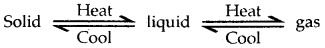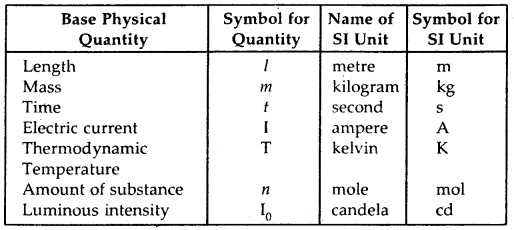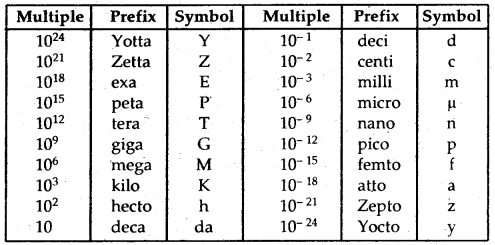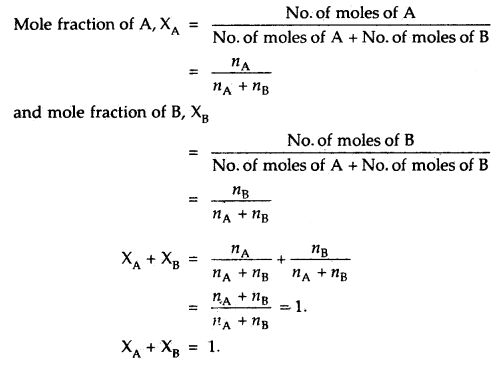By going through these CBSE Class 11 Chemistry Notes Chapter 1 Some Basic Concepts of Chemistry, students can recall all the concepts quickly.

## Some Basic Concepts of Chemistry Notes Class 11 Chemistry Chapter 1

Importance of Chemistry: Chemistry plays a central role in science and is often intertwined with branches of science like Physics, Biology, Geology, etc. Chemistry also plays an important role in meeting human needs for food, health care products, etc.

→ Chemistry deals with the composition, structure, and properties of matter. To understand Chemistry, we have to study the basic constituents of matter Atoms & Molecules and their relationship with mass. Chemistry is basically, about chemical transformations.

→ Different manifestations of Chemistry include fertilizers, alkalies, acids, salts, dyes, polymers, drugs, soaps, detergents, metals, alloys, and other inorganic and organic chemicals. Daily new drugs, dyes, polymers, etc. are finding their way from the laboratory to industry. Many life-saving drugs like Cisplatin and Taxol are proving effective in cancer therapy and AZT [Azidothymidine] in helping Aids victims.

Nature of Matter: Anything which has mass and occupies space is called matter. Matter can exist in three physical states-solids, liquids, and gases.

→ Solids: Solids have definite volume and definite shape.

→ Liquids: Liquids have a definite volume, but no definite shape. They take the shape of the container in which they are put.

→ Gases: Gases have neither definite volume nor definite shape. These three forms are interconvertible.Matter can be classified as mixtures and pure substances. Mixtures can be both homogeneous as well as heterogeneous. Milk, air are examples of homogeneous mixtures. They are uniform throughout. If they are not having a uniform composition, they are a heterogeneous mixture. Iron and sand is an example of a heterogeneous mixture.

Elements like Cu, Ag, and compounds like Nad, AgNO, constitute pure substances. Elements consist of only one type of particle. When two or more elements combine, they form compounds. The smallest part of an element is an Atom whereas the smallest particle of a compound is a Molecule. Thus, copper is an element composed of Cu atoms whereas sodium chloride is a compound composed of Na+Cl molecules.Properties of Matter and their Measurement: Properties can be classified into two categories:

1. Physical properties and
2. Chemical properties.

→ Physical properties: Physical properties are those which can be observed or measured without changing the identity or composition of the substance. Some examples of physical properties are color, odor, melting point, boiling point density, etc.

The measurement or observation of chemical properties requires a chemical change to occur. Examples of chemical properties are aridity, basicity, combustibility.

The molar mass calculator with steps uses the chemical formula to determine the number of atoms of each element in the compound.

Base Physical Quantities and their Units:Definitions of SI Base Units:In SI, large and small quantities are expressed by using an appropriate prefix with the base units.

S.I. Prefixes:Mass of a substance is the amount of matter present in it while weight is the force exerted by gravity on an object. The mass of a substance is constant whereas its weight may vary from one place to another due to change in gravity.
The SI unit of mass is a kilogram However, its fraction gram (1 kg = 1000 g), is used in laboratories due to the smaller amounts of chemicals used in chemical reactions.

Volume has the units of (length)3. So in the SI system, the volume has units of m3. But again, in Chemistry laboratories, the smallest volumes are used. Hence volume is often denoted by cm3 or dm3 units. A common unit, liter (L) which is not a SI unit, is used for the measurement of the volume of liquids.
1 L = 1000 mL, 1000 cm3 = 1 dm3

→ Density: Density of a substance is its amount of mass per unit volume.
∴ SI unit of density = $$\frac{\text { SI unit of mass }}{\text { SI unit of volume }}$$
= $$\frac{\mathrm{kg}}{\mathrm{m}^{3}}$$ = kg m-3
This unit is very large.

∴ Density is usually expressed is g cm-3.

→ Temperature: SI unit of temperature is kelvin (K)
K = °C + 273.15
where °C is degree Celsius.

It is interesting to note that temperatures below 0°C (i.e., negative values) are possible on the Celsius scale but, in the Kelvin scale, the negative temperature is not possible.

In addition to Kelvin (K – SI unit), there are two more scales to measure temperature.

1. Celsius scale (°C)
2. Fahrenheit scale (°F)
°F = $$\frac{9}{5}$$(°C) + 32

Uncertainty in Measurement: Many a time in the study of Chemistry, one has to deal with experimental data as well as theoretical calculations. There are meaningful ways to handle the numbers conveniently and present the data in a realistic way with certainly to the extent possible.

→ Scientific Notation: We can write 232.508 as 2.32508 × 102 in scientific notation. Similarly, 0.00016 can be written as 1.6 × 10-4.

→ Multiplication and Division
Multiplication: (5.6 × 105) × (6.9 × 108) = (5.6 × 6.9)(105 + 8)
= 5.6 × 6.9 × 1013
= 38.64 × 1013

Division:
$$\frac{2.7 \times 10^{-3}}{5.5 \times 10^{4}}$$ = (2.7 ÷ 5.5)(10-3-4)
= 0.4909 × 10-7

Addition: 6.65 × 104 + 8.95 × 103
= 6.65 × 104 + 0.895 × 104
= (6.65 + 0.895) × 104
= 7.545 × 104

Subtraction:2.5 × 10-2 – 4.8 × 10-3
= 2.5 × 10-2 – 0.48 × 10-2
= (2.5-0.48)10-2
= 2.02 × 10-2

→ Significant Figures: All experimental measurements have some degree of uncertainty associated with them. Precision and accuracy are often referred to while we talk about measurement.

→ Precision: Precision refers to the closeness of various measurements for the same quantity. However, accuracy is the agreement of a particular value to the true value of the result.

→ Significant figures: Significant figures are meaningful digits that are known with certainty.

→ Dimensional Analysis: Often in calculations, We have to convert units from one system to another. The method used to accomplish this is called the factor label method or unit factor method or dimensional analysis.

→ Laws of Chemical Combination: The combination of elements to form compounds is governed by the following five basic laws.

1. Law of Conservation of Mass
2. Law of Definite Proportions
3. Law of Multiple Proportions
4. Gay Lussac’s Law of Gaseous Volumes

1. Law of conservation of mass: In all physical and chemical changes, the total mass of the reactants is equal to that of the products.
Or
Matter can neither be created nor destroyed.

2. Law of definite proportions or Law of constant composition: The law states “A chemical compound always consists of the same elements combined together in the same fixed proportion by weight”.

3. Law of multiple proportions: When two elements combine to form two or more than two compounds, then the weights of the elements which combine with the fixed weight of the other, bear a simple ratio to one another.

4. Gay-Lussac’s Law of Gaseous Volumes: It states “When gases react together, they always do so in volumes which bear a simple ratio to one another and to the volumes of the products if gaseous, provided all measurements of volumes are done under similar conditions of temperature and pressure”.

5. Avogadro’s Hypothesis Or Law: Instates, “Equal volumes of all gases under similar conditions of temperature and pressure contain an equal number of molecules”.

Dalton’s Atomic Theory: The main postulates of this theory are:

1. Matter consists of indivisible atoms.
2. All the atoms of a given element have identical properties including identical mass. Atoms of different elements differ in mass.
3. Compounds are formed when atoms of different elements combine in a fixed ratio.
4. Chemical reactions involve the reorganization of atoms. These are neither created nor destroyed in a chemical reaction.

The main advantage of Dalton’s theory was that it could explain the laws of chemical combination.

Atomic and Molecular Masses:
1. Atomic Mass: The atomic mass of an element is the number of times an atom of that element is heavier than an atom of carbon taken as 12.

→ Atomic Mass Unit,(AMU) or u: One atomic mass unit (AMU) or u is equal to $$\frac{1}{12}$$th the mass of an atom of carbon – 12 isotopes.

1 amu = 1.66056 × 10-24 g
Mass of an atom of hydrogen = 1.6736 × 10-24 g

Thus, in terms of amu,
the mass of hydrogen atom = $$\frac{1.6736 \times 10^{-24} \mathrm{~g}}{1.66056 \times 10^{-24} \mathrm{~g}}$$
= 1.0078 amu = 1.0080 amu

Similarly, the mass of oxygen – 16(16O) atom would be 15.995 amu

Today, ‘amu’ has been replaced by u which is known as unified mass.

→ Average Atomic Mass: From different isotopes of an element based on their relative abundance, the average atomic mass of an element can be calculated.

For example, there are three isotopes of C – (12C, 13C, 14C) with their relative abundance (%) – 98.892, 1.108, and 2 × 10-10 with their atomic mass (AMU) as 12, 13.00335, and 14.00317 respectively.
The average atomic mass of carbon will be
=.(0.98892)(12 u) + (0.01108)(13.00335 u) + (2 × 10-12)(14.00317 u)
= 12.011 u.

→ Molecular Mass: Molecular mass is the sum of atomic masses of the elements present in a molecule.

Molecular mass of water (H2O)
= 2 × atomic mass of hydrogen + 1 × atomic mass of oxygen = 2(1.008 u) + 16.00 u = 18.02 u,

Molecular mass of ethane (C2H6) will be = 2 × (12,011 u) + 6 × (1 008 u)
= 24.022 u + 6.048 u = 30 070 u

→ Formula Mass: The formula mass of NaCl which is composed of Na+ and Cl ions in a three-dimensional structure can be calculated
= Atomic mass of sodium + Atomic mass of chlorine
= 23.00 u + 35.5 u = 56.5 u.

Mole Concept and Molar Masses: In the SI system, mole (symbol, mol) was introduced as the base quantity for the amount of a substance.

→ Substance One Mole is the amount of a substance that contains as many particles or entities as there are atoms in exactly 12 g (or 0. 012 kg) of the 12C Isotope.

1 Mole = 6.0221367 × 1023 atoms
The number of entities in 1 mol is also called Avogadro Constant and denoted by NA.

Therefore, 6:022 × 1023 entities (atoms, molecules, or any other particles) constitute one mole of that particular substance.
∴ 1 Mol of oxygen atoms = 6.022 × 1023 atoms of oxygen.

Similarly, 1 Mol of ammonia molecules
= 6.022 × 1023 molecules of ammonia
where n maybe 1, 2, 3,…..

In the SI system, Mole (symbol, mol) was introduced as the seventh base quantity for the amount of a substance.

One mole is the amount of a substance that contains as many particles or entities as there are atoms in exactly 12g (or 0.012 kg) of the UC isotope.

Stoichiometry and Stoichiometric Calculations: Stoichiometry deals with the calculation of masses and volumes of the reactants and products involved in a chemical reaction.

Let us consider the information available for the combustion of methane from its balanced chemical equation
CH4(g) + 2O2(g) → CO2(g) + 2H2O(g)

Here methane and dioxygen are called reactants and carbon dioxide and water are called products. The letter (g) stands for gases. The coefficient 2 for O2 and H2O are called stoichiometric coefficients. Similarly, the coefficient for CH4 and CO2 is one in each case.

They represent the number of molecules (and moles as well taking part in the reaction or formed in the reaction).
(a) One mole of CH4(g) reacts with two moles of O2(g) to give one mole of CO2(g) and two moles of H2O(g).
(b) One molecule of CH4(g) reacts with 2 molecules of O2(g) to give one molecule of CO2(g) and 2 molecules of H2O(g).
(c) 22.4 L of CH4(g) reacts with 44.8 L of O2(g) at STP to give 22.4 L of CO2(g) and 44,8 L of H2O (g).
(d) 16 g of CH4(g) reacts with 64 g of O2(g) to give 44 g of CO2(g) and 36 g of H2O(g)

From above
mass ⇌ moles ⇌ no. of molecules
$$\frac{\text { Mass }}{\text { Volume }}$$ = Density

→ Limiting Reagent: The reacting substance which gets used up first in the reaction is called the limiting reagent. This is because the amount of limiting reagent limits the amount of the product formed. A part of the other reactants which are present in amounts greater than the stoichiometric amounts is left behind as unconsumed reagents.

→ Reactions in Solutions: The concentration of a solution or the amount of substance present in its given volume can be expressed in any of the following ways:

1. Mass percent or weight percent (w/w%)
2. Mole fraction
3. Molarity
4. Molality

Stoichiometry of Reactions in Solutions:
1. Mass percentage or percent by mass: It is defined as the mass of solute in gram per 100 g of the solution. For example, a 10% solution of sodium chloride means that 10 g of NaCl is present in 100 g of the solution.

Mass % of the solute = $$\frac{\text { Mass of solute }}{\text { Mass of solution }}$$ × 100
Both, the mass of the solute and that of the solution must be expressed in the same mass units. viz, both in grams or both in
kilograms, etc.

2. Mole Fraction (X): The mole fraction of any component of a solution is defined as the ratio of the number of moles of that component to the total number of moles of all the components of the solution. Thus if a solution contains A moles of A and n5 moles of B, then.If the mole fraction of one component in a binary solution is known, that of the other can be determined.
i.e. XB = 1 – XA.

3. Molarity (M): The molarity of a solution is defined as the number of moles of solute dissolved per dm3 (or liter, L) of the solution. Molarity of any solution depends upon temperature. So, the molarity of any solution is specified for a given temperature.

Mathematically, molarity is defined as
Molarity of solution = $$\frac{\text { No. of moles of the solute }}{\text { Volume of the solution in litres (or in } \mathrm{dm}^{3} \text { ) }}=\frac{n \mathrm{~mol}}{\mathrm{VL}}$$

where n is the number of moles of the solute.
V is the volume of the solution in liters (or dm3).

Since, the number of moles of any substance is related to its mass and the molar mass,
No. of moles of solute = $$\frac{\text { Mass of the solute }}{\text { Molar mass of the solute }}$$

The molarity of a solution then can also be expressed as:
Molarity of the solution = $$\frac{\text { Mass of the solute }}{\text { Molar mass of the solute } \times \text { Volume of the solution in litres }}$$

So, W grams of a substance having molar mass M, dissolved in sufficient solvent, so as to make the total volume of V liter of the substance, the molarity (M) of the solution is given by
Molarity = $$\frac{\mathrm{Wg}}{\mathrm{Mg} \mathrm{mol}^{-1} \times \mathrm{V} \text { litre }}=\frac{\mathrm{W}}{\mathrm{M} \times \mathrm{V}}$$ mol/L

4. MoLdity (m): The molality of a solution is defined as the number of moles of solute per kg of the solvent. If a solution is prepared by dissolving n moles of a solute in W kg of the solvent, then.
Molality, (m) = $$\frac{\text { No. of moles of solute }}{\text { Mass of the solvent in } \mathrm{kg} \mathrm{mol}^{-1}}$$

= $$\frac{n_{\text {solute }}}{W_{\text {solvent }} \text { kg }}=\frac{n_{\text {solute }}}{W_{\text {solvent }}}$$mol kg-1
where nsolute = $$\frac{\text { Mass of the solute }}{\text { Molar mass of the solute }}$$

It is to be noted that molality of a solution does not change with temperature since mass remains unaffected with temperature.

→ Element: It consists of only one type of particles. These particles may be atoms or molecules. Sodium, copper, silver, hydrogen, oxygen etc. are some examples of elements.

→ Compound: When two or more atoms of different elements combine in a fixed proportion, the molecules of a compound is obtained.

→ Atomic mass unit (amu): It is defined as a mass exactly equal to one twelfth the mass of one carbon-12.
1 amu = 1.66056 × 10-24 g
Nowadays’ amu’ has been replaced by u which is known as unified mass.

→ Mole: One mole is the amount of a substance that contains as many particles or entities as there are atoms in exactly 12 g {or 0.012 kg) of the 12C isotope.

→ Avogadro constant: The number of entities in 1 mol is called the Avogadro constant. It is denoted by NA and is = 6.022 × 1023.

→ Molar mass: The mass of one mole of a substance in grams is called its molar mass.

→ Empirical Formula represents the simplest whole-number ratio of various atoms present in a compound.

→ Molecular Formula: It shows the exact number of different types of atoms present in a molecule of a compound.

→ Mass percent:
Mass per cent = $$\frac{\text { Mass of solute }}{\text { Mass of solution }}$$ × 100

→ Mole Fraction:
Mole fraction of a component = $$\frac{\text { No. of moles of the component }}{\text { Total no. of moles of solution }}$$

→ Molarity:
Molarity(M) = $$\frac{\text { No. of moles of the solute }}{\text { Volume of solution in litres }}$$

→ Molality
Molality (m) = $$\frac{\text { No. of moles of solute }}{\text { Mass of solvent in } \mathrm{kg}}$$

→ There are different laws which govern the combination of elements to form compounds.
They are mentioned below:

1. Law of conservation of mass.
2. Law of Definite Proportions.
3. Law of multiple proportions.
4. Gay Lussac’s Law of gaseous Volumes.